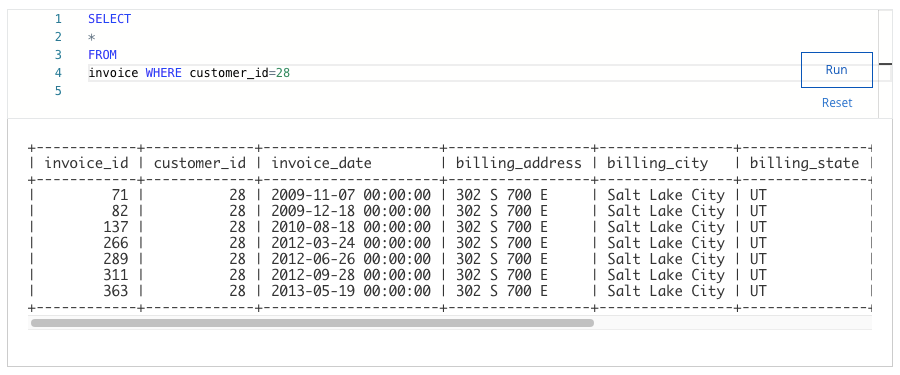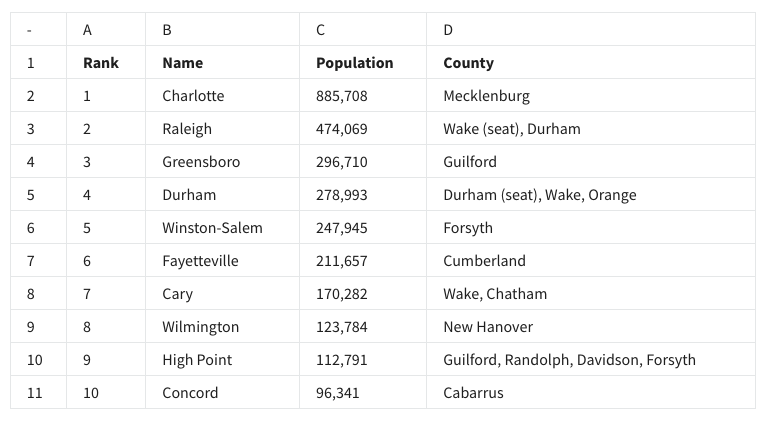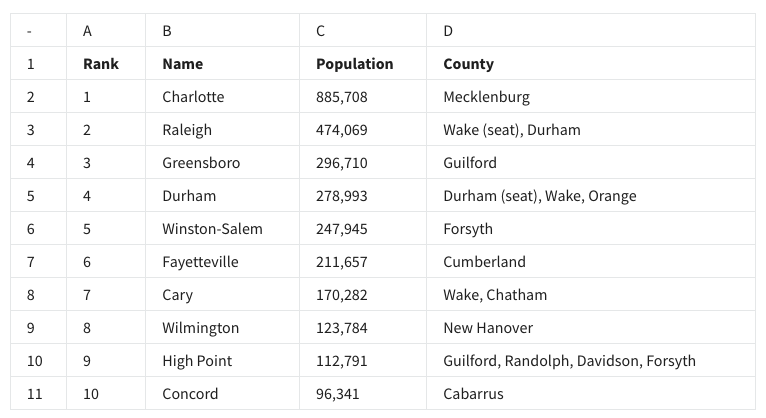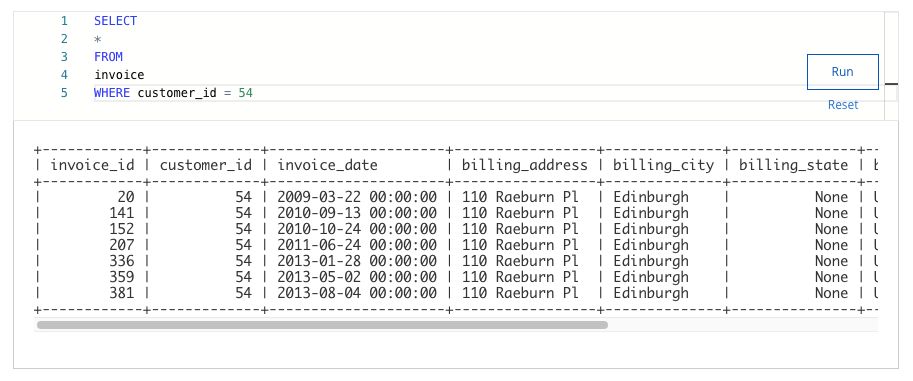## Week 4 – Set up your toolbox – Shuffle Q/A 2

#### 21. You are working with a database table that contains invoice data. The customer_id column lists the ID number for each customer. You are interested in invoice data for the customer with ID number 28. You write the SQL query below. Add a WHERE clause that will return only data about the customer with ID number 28.#### After you run your query, use the slider to view all the data presented.What is the billing city for the customer with ID number 28?

• Bangalore
• Buenos Aires
• Dijon
• Salt Lake City

#### 22. Which of the following best describes a bar chart?

• It is a visualization that uses a circle which is divided into wedges sized based on numerical proportion.
• It is a visualization that plots a sequence of points and connects them with them with straight lines or curves.
• It is a visualization that represents data with columns, or bars, the heights of which are proportional to the values that they represent.
• It is a visualization that plots individual points in the Cartesian coordinate plane.

#### 23. A data analyst has to create a visualization that clearly shows when and for how long the population of Charlotte has been above one million people. They choose to use a line chart. Why is this the best choice for their visualization?

• It is a visualization that plots a sequence of points and connects them with straight lines or curves.
• It is a visualization that uses a circle which is divided into wedges sized based on numerical proportion.
• It is a visualization that represents data with columns, or bars, the heights of which are proportional to the values that they represent.
• It is a visualization that plots individual points in the Cartesian coordinate plane.

#### 24. The words rank, name, population, and county in row 1 of the following spreadsheet are known as descriptors.• True
• False

#### 25. Fill in the blank: In the following spreadsheet, the ________ of High Point describes all of the data in row 10.• criteria
• dataset
• observation
• format

#### 26. If a data analyst wants to list the cities in this spreadsheet alphabetically, instead of numerically, what feature can they use in column B?• Sort range
• Name range
• Randomize range
• Organize range

#### 27. A data analyst wants to create a visualization that depicts the populations of the top ten most populous cities in North Carolina. What type of chart would be best for this?

• A pie chart
• A scatter chart
• A column, or bar, chart
• A line chart

• Line
• Area
• Bar
• Column

## Shuffle Q/A 3

#### 29. You are working with a database table that contains invoice data. The customer_id column lists the ID number for each customer. You are interested in invoice data for the customer with ID number 54.You write the SQL query below. Add a WHERE clause that will return only data about the customer with ID number 54.#### After you run your query, use the slider to view all the data presented.What is the billing address for the customer with ID number 54?

• 1033 N Park Ave
• 230 Elgin St
• 110 Raeburn Pl
• 801 W 4th St

• chart editor
• rename
• filter view
• image